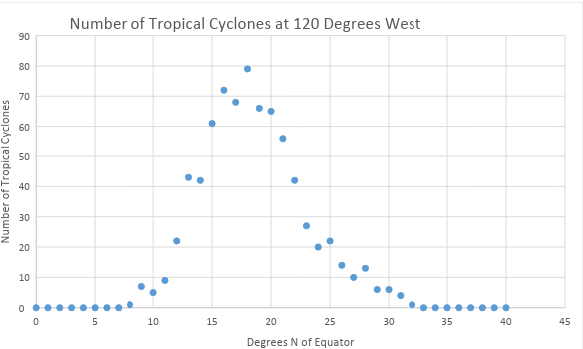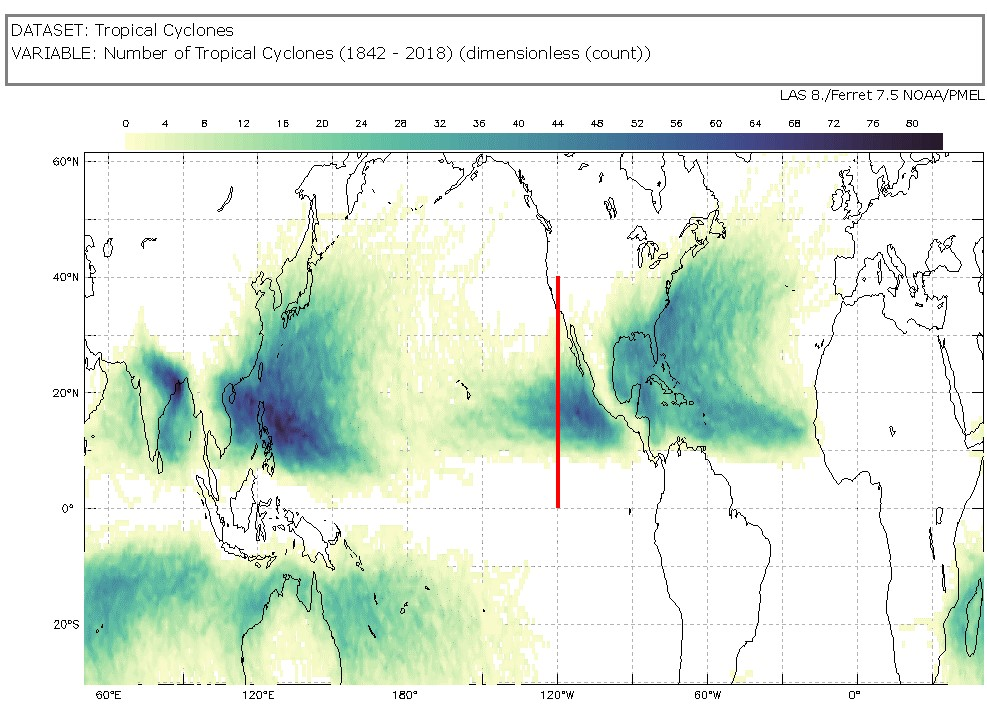# Tropical Cyclone Counts Scatter Plot

### Overview

Interpret a scatter plot to find patterns in the number of tropical cyclones from 1842 to 2018.

### Student Directions

Tropical cyclones are sometimes called hurricanes or typhoons. These data may be visualized in a variety of ways.

Below you will find two types of visualizations of the same data, a scatter plot and a map. You will analyze these and extract information. Recall, a scatter plot is a type of graph which shows a relationship, or correlation, between two variables. One variable is on the x axis and the other is on the y axis. One may use a scatter plot to answer specific kinds of questions about their data.

• What is the relationship between the two variables?
• Does one variable increase when the other variable decreases?
• Does one variable increase when the other variable increases?
• Is there a correlation between the two variables?
• Is there a positive, negative or no relationship between the variables?

#### Steps

1. The Number of Tropical Cyclones at 120 Degrees West Scatter Plot shows the number of tropical cyclones at 120° west for each degree of latitude from the equator (0°) to 40° north; the same as represented along the red line in the mapped image below.Scatter plot - number of tropical cyclones at 120 degrees west https://mynasadata.larc.nasa.gov/sites/default/files/inline-images/thumbnail.png
1. What does the scatter plot show? What does the scatter plot NOT show?
2. Is the plot linear (do the points appear to lie close together along a straight line) or nonlinear (do the points appear to form a curve)?
3. The image shows the number of tropical cyclones around the world from 1842 – 2018. There is a thick line at 120 degrees west from the equator to 40 degrees north; the same as represented by the scatter plot. The Tropical Cyclone Counts map was generated in the My NASA Data Earth System Data Explorer. Now compare the scatter plot to the map image and answer the following questions.Tropical Cyclone Counts Map showing a line at 120 degrees west from the equator to 40 degrees north https://mynasadata.larc.nasa.gov/sites/default/files/2022-02/Tropical%20Cyclone%20count%20120%20W.png
1. Which visualization, the scatter plot, map or both, shows the number of tropical cyclones at each latitude?
2. Which data visualization, the scatter plot or map, best helps you answer questions about specific number of tropical cyclones at specific locations?
3. Which data visualization, the scatter plot or map, best helps you answer questions about tropical cyclones around the world?

### Teacher Note

Teachers, these mini lessons/student activities are perfect "warm up" tasks that can be used as a hook, bell ringer, exit slip, etc. They take less than a class period to complete. Learn more on the "My NASA Data What are Mini Lessons?" page.

Teachers who are interested in receiving the answer key, please complete the Teacher Key Request and Verification Form. We verify that requestors are teachers prior to sending access to the answer keys as we’ve had many students try to pass as teachers to gain access.

• 6-8

• 30 minutes